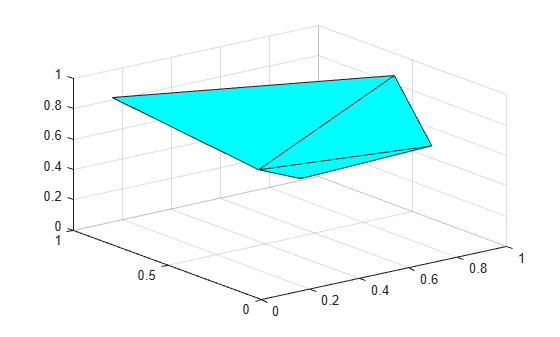convhulln

N-D convex hull

Description

example

k = convhulln(P) computes the N-D convex hull of the points in a matrix P.

k = convhulln(P,opts) also specifies the Qhull options used to compute the convex hull.

example

[k,vol] = convhulln(___) also returns the volume vol of the convex hull for either of the previous syntaxes.

Examples

collapse all

Create a matrix of 3-D points and compute the convex hull and its volume.

P = rand(10,3);
[k,vol] = convhulln(P);

Plot the convex hull.

trisurf(k,P(:,1),P(:,2),P(:,3),'FaceColor','cyan')Display the volume of the convex hull.

vol
vol = 0.1857

Input Arguments

collapse all

Points, specified as a matrix whose columns contain the coordinates for the corresponding dimension. For example, to define a set of 2-D points, place the x-coordinates in the first column of P and the corresponding y-coordinates in the second column.

Qhull options, specified as a cell array of character vectors indicating which Qhull algorithms to use. For a list of options, see Qhull control options.

By default, opts is set to {'Qt'} for 2-, 3-, and 4-dimensional input. For 5-dimensional input and higher, opts is set to {'Qt','Qx'}.

Output Arguments

collapse all

Indices, returned as a vector or matrix.

• For 2-D points, k is a column vector containing the row indices of the input points that make up the convex hull, arranged counterclockwise.

• For 3-D points, k is a three-column matrix where each row represents a facet of a triangulation that makes up the convex hull. The values represent the row indices of the input points.

• For N-D points, k is an N-column matrix. The values represent the row indices of the input points.

Volume of the convex hull (area for two dimensions), returned as a scalar.

Algorithms

convhulln is based on Qhull . For information, see http://www.qhull.org/. For copyright information, see http://www.qhull.org/COPYING.txt.

 Barber, C.B., D.P. Dobkin, and H.T. Huhdanpaa. “The Quickhull Algorithm for Convex Hulls.” ACM Transactions on Mathematical Software, Vol. 22, No. 4, Dec. 1996, p 469–483.